Go back to  'Triangles'

The angles and lines of a triangle play a major role in the construction of it.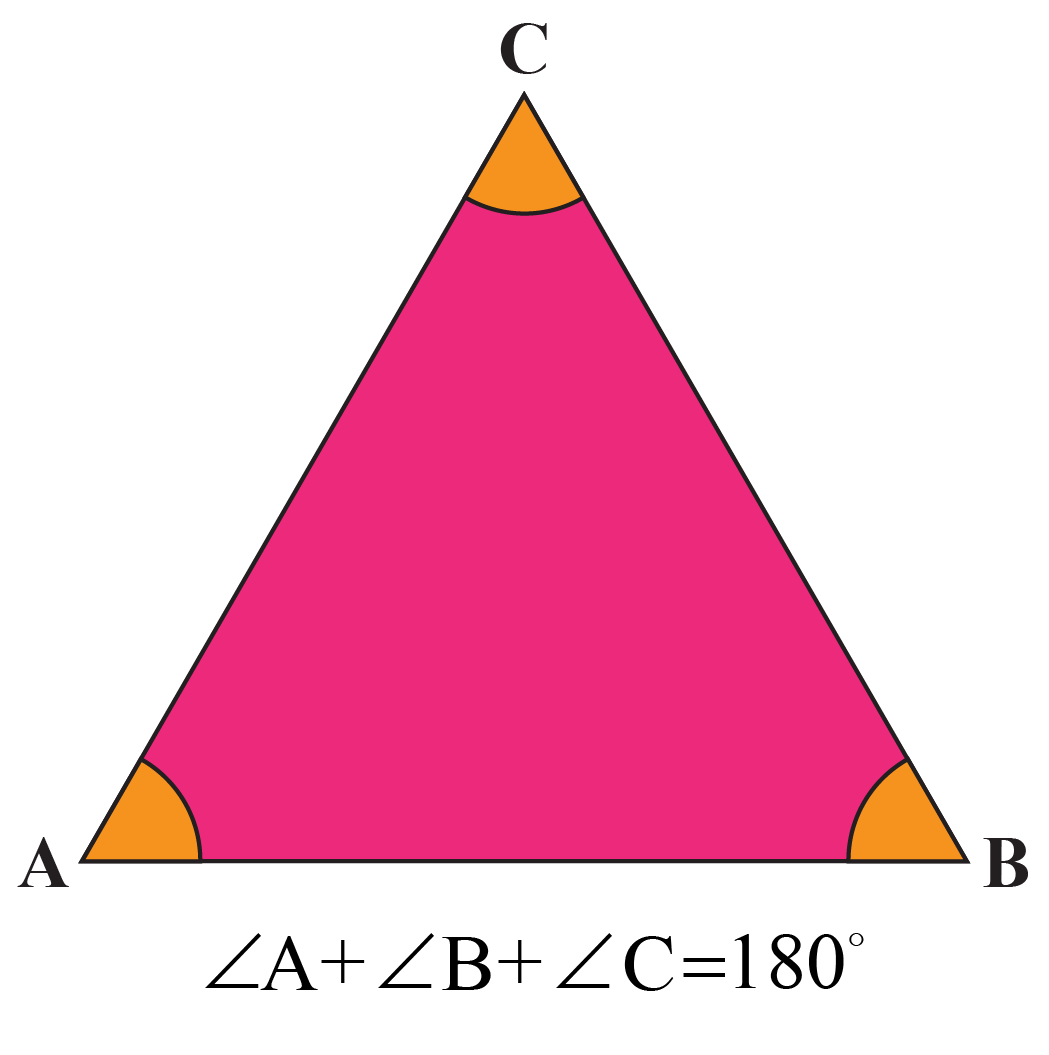## What Are the Types of Triangles?

Triangles can be classified on the basis of:

1) Angles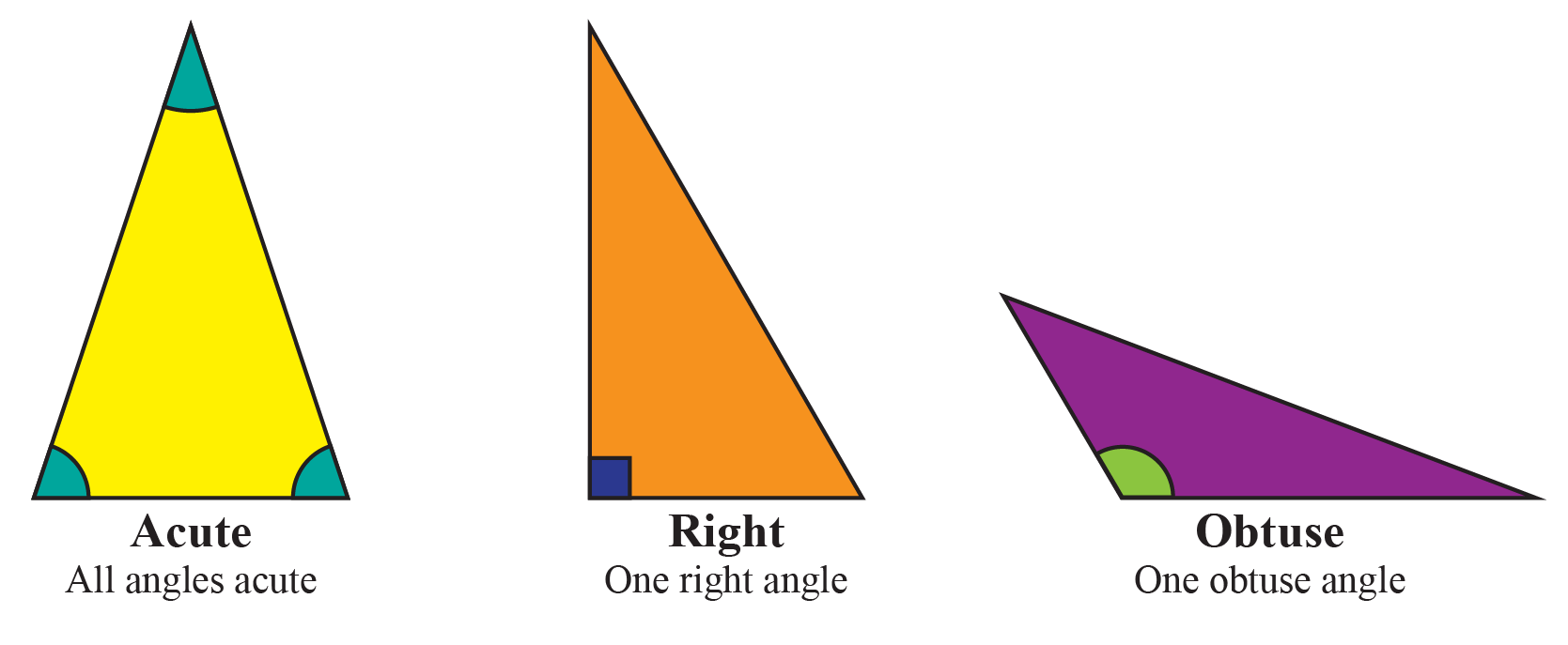2) Sides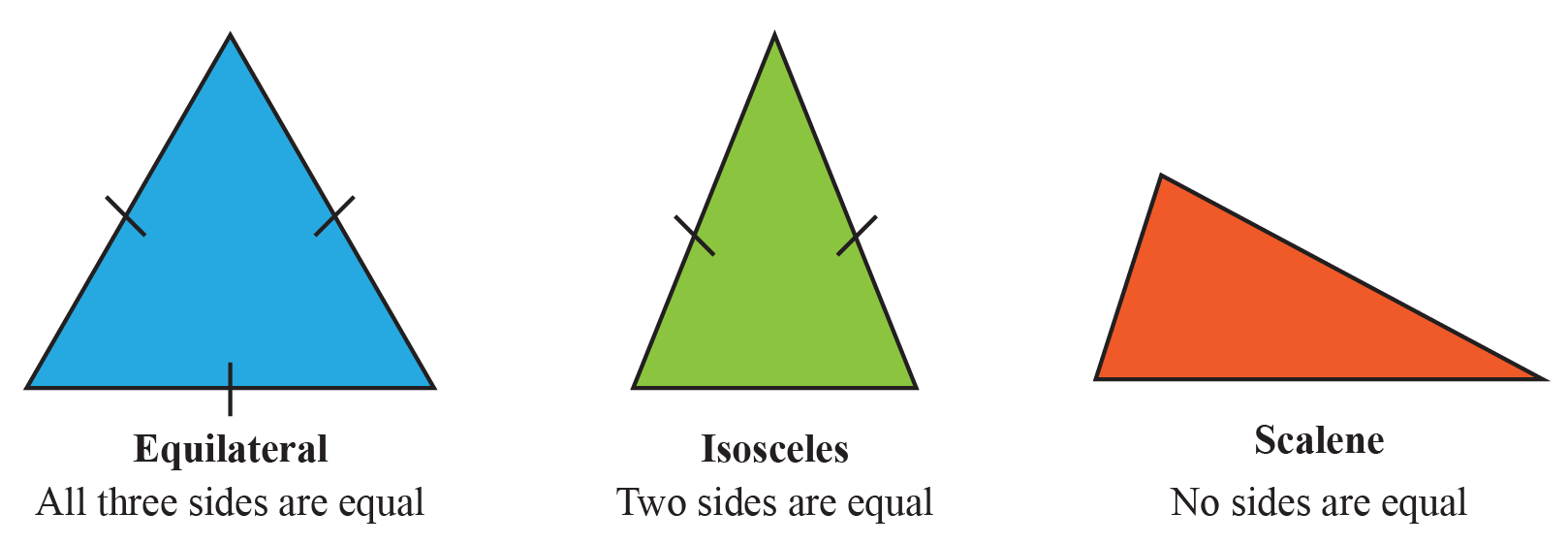3) Angles and Sides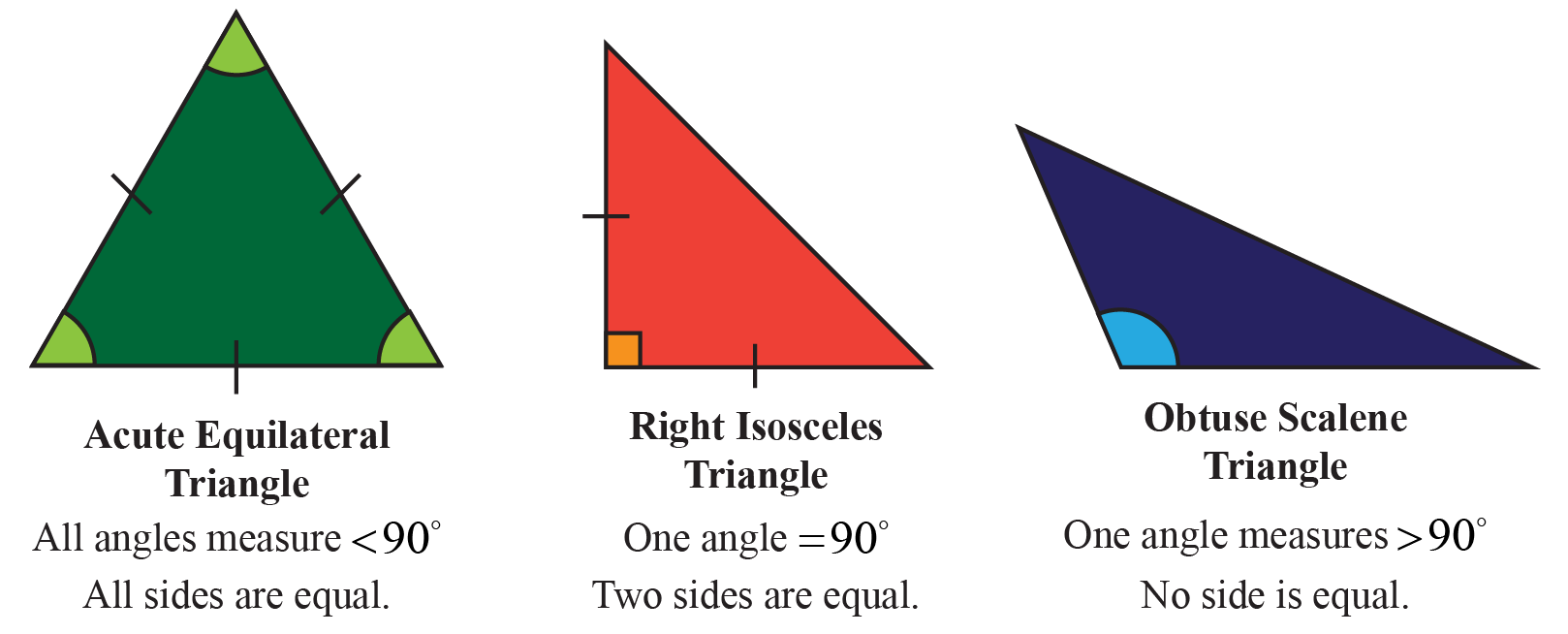## Methods for Construction of a Triangle

We are going to construct $$\Delta ABC$$, in which:

### Method 1

The base is BC, base angle $$\angle B$$, and AB + AC are given.

Step 1: Draw the base BC, and through B, draw a ray BX inclined at the angle specified: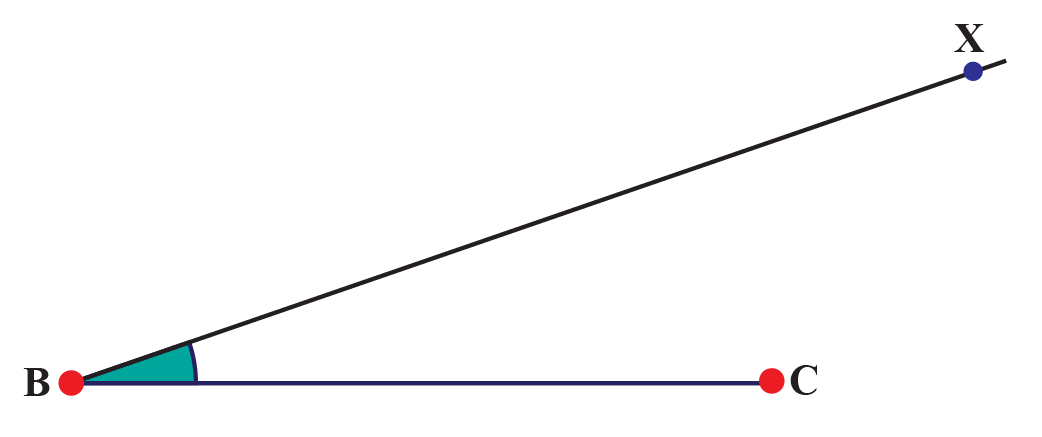Step 2: On BX, cut off BD equal to AB + AC: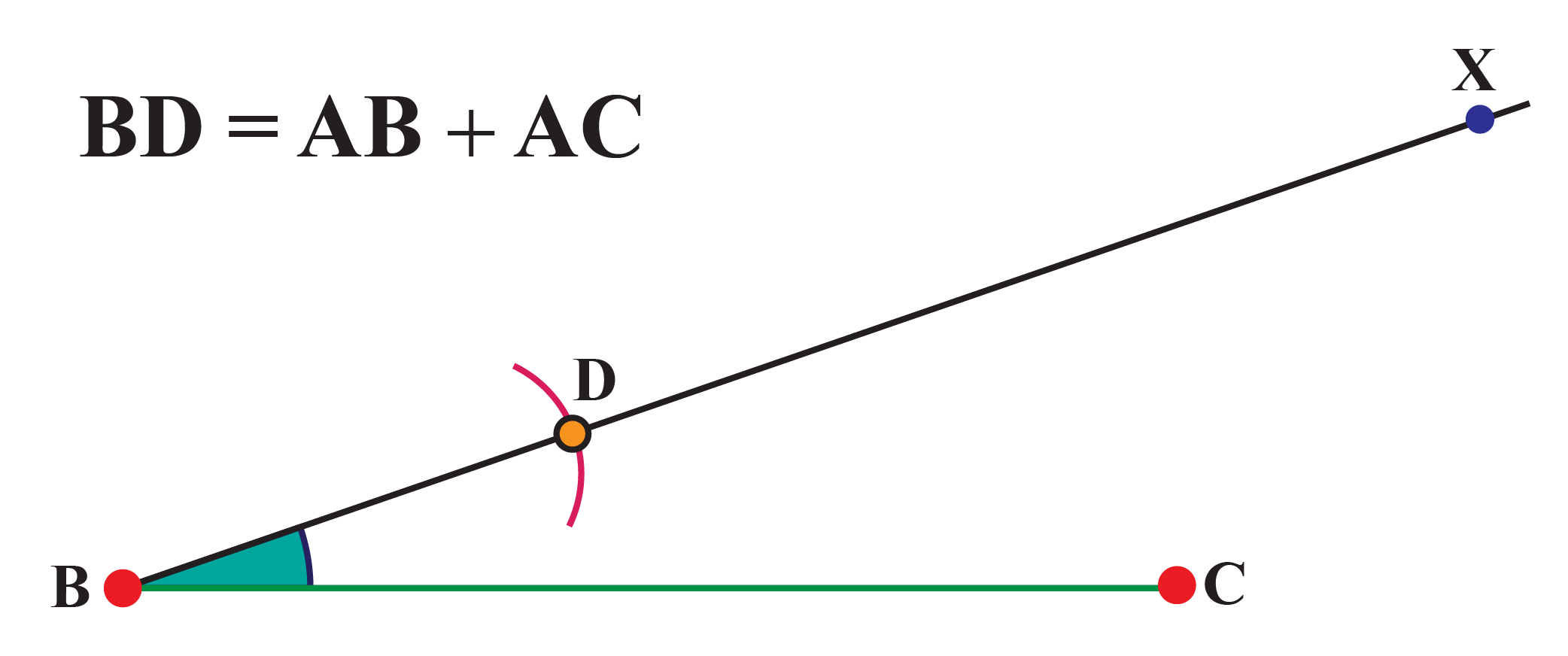Step 3: Join CD, and draw a ray CY such that $$\angle DCY$$= $$\angle BDC$$. Let that ray intersect the ray BX at A: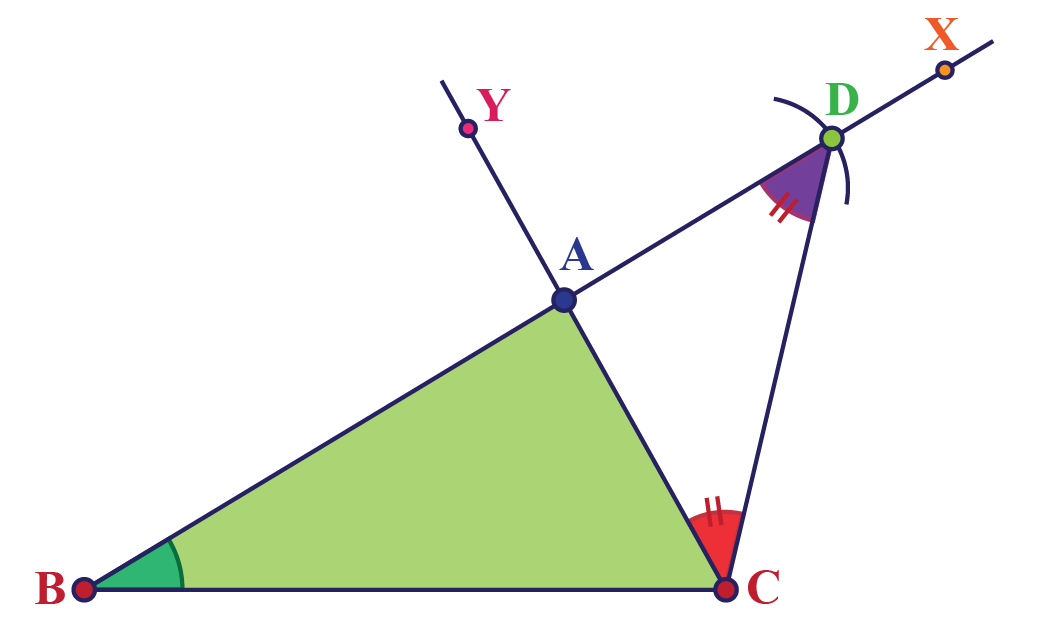$$\Delta ABC$$ is the required triangle. This is because in $$\Delta ACD$$, we will have AC = AD (due to our construction such that the opposite angles $$\angle DCY$$and $$\angle BDC$$are equal). Thus,

AB + AD = AB + AC

Alternatively, after Step 2, you could have drawn the perpendicular bisector of CD. The point of intersection of this perpendicular bisector with ray BX would also have given you point A: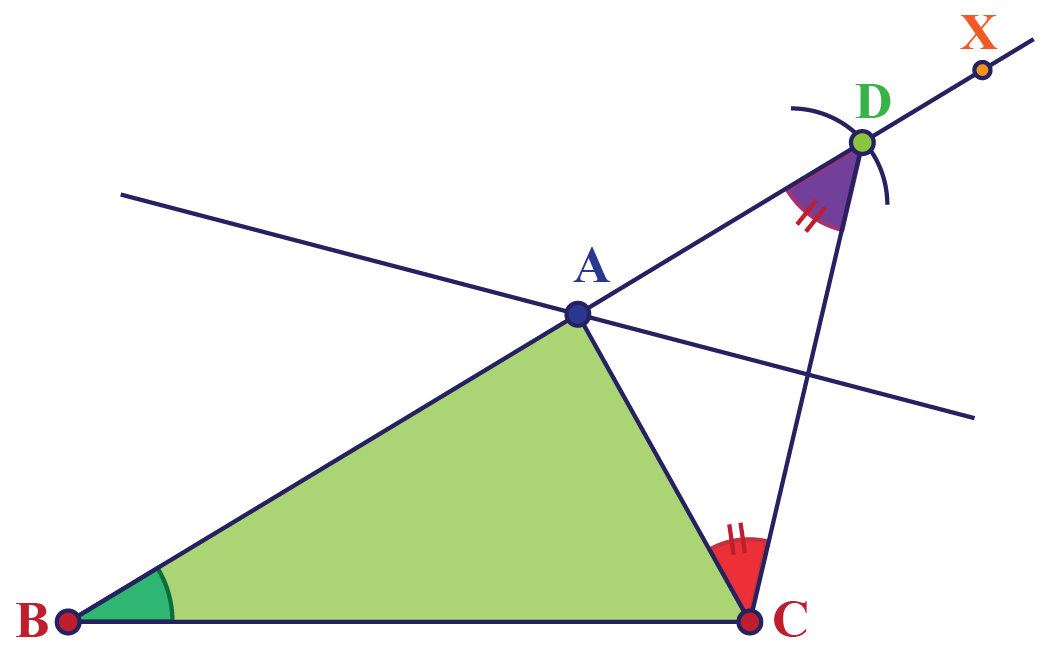This is because since A lies on the perpendicular bisector of CD, we will have AC = AD, and again:

AB + AD = AB + AC

### Method 2

The base BC is given, a base angle is given, and the difference between the other two sides is given.

We will construct $$\Delta ABC$$, given BC, $$\angle B$$, and the difference between AB and AC. Note that the difference between AB and AC does not specify which of the two will be larger. For example, if you are given that the difference between x and y is 3, there are two cases: x – y = 3, or y – x = 3. Therefore, we will have to consider two cases, one in which AB is larger, and one in which AC is larger.

### Case 1: AB > AC

The steps of the construction will be as follows:

Step 1: Draw BC, and draw a ray BX through B which is inclined to BC at the given angle value:Step 2: On BX, cut off BD equal to AB – AC: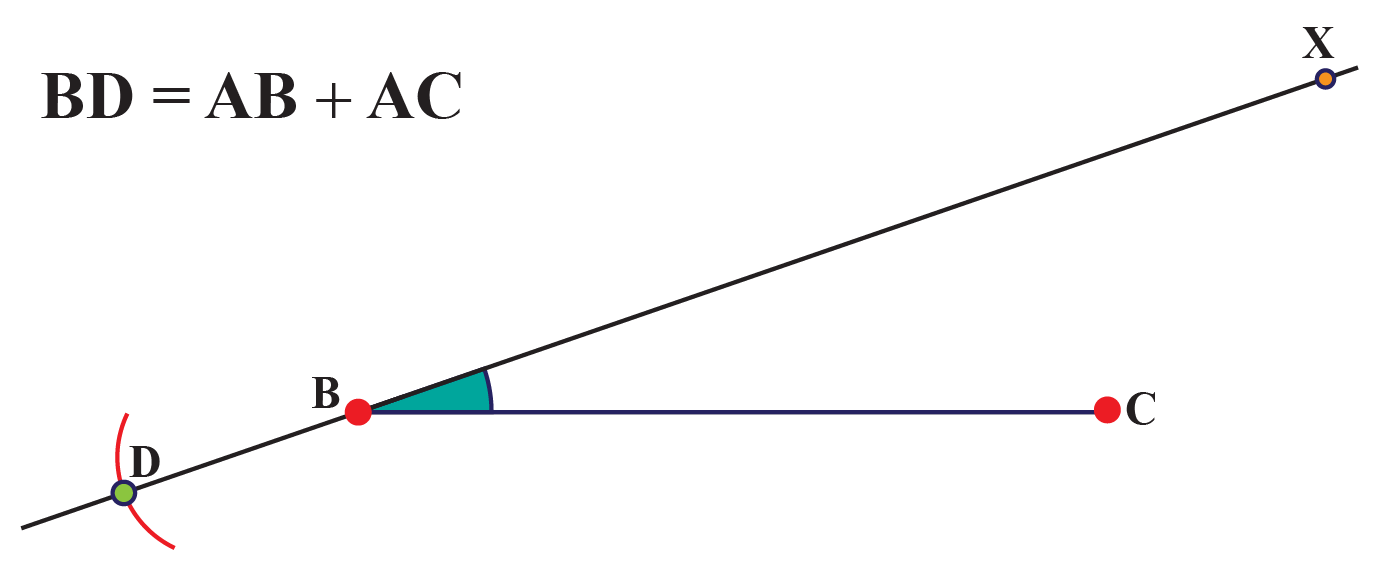Step 3: Join CD and draw the perpendicular bisector of CD. Let it intersect ray BX at A: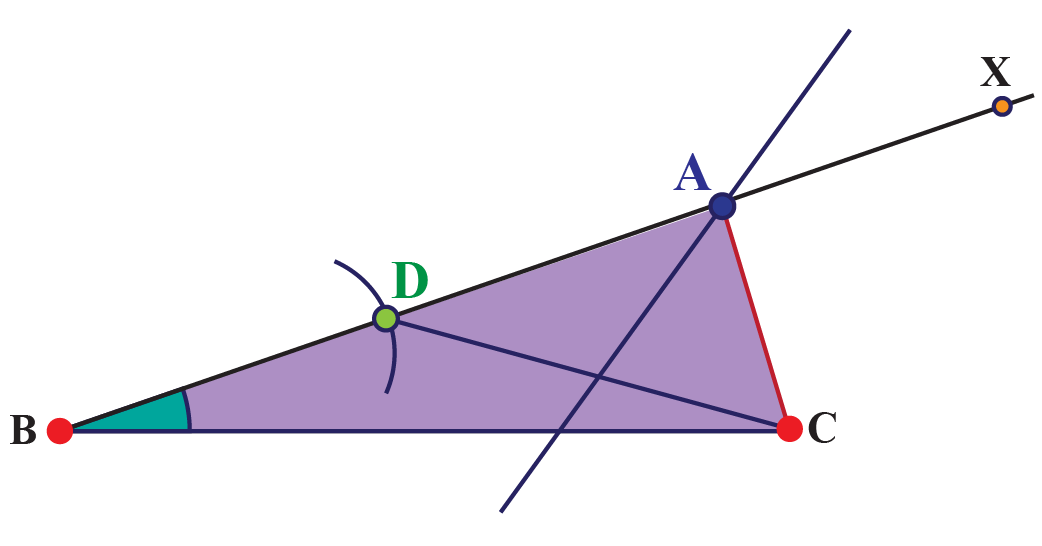$$\Delta ABC$$is the required triangle, because AC = AD (why?), and thus,

BD = AB – AD = AB – AC

### Case 2: AC > AB

The first step is the same (drawing BC and ray BX inclined to BC at the specified angle, but now, extend BX on the opposite side as well: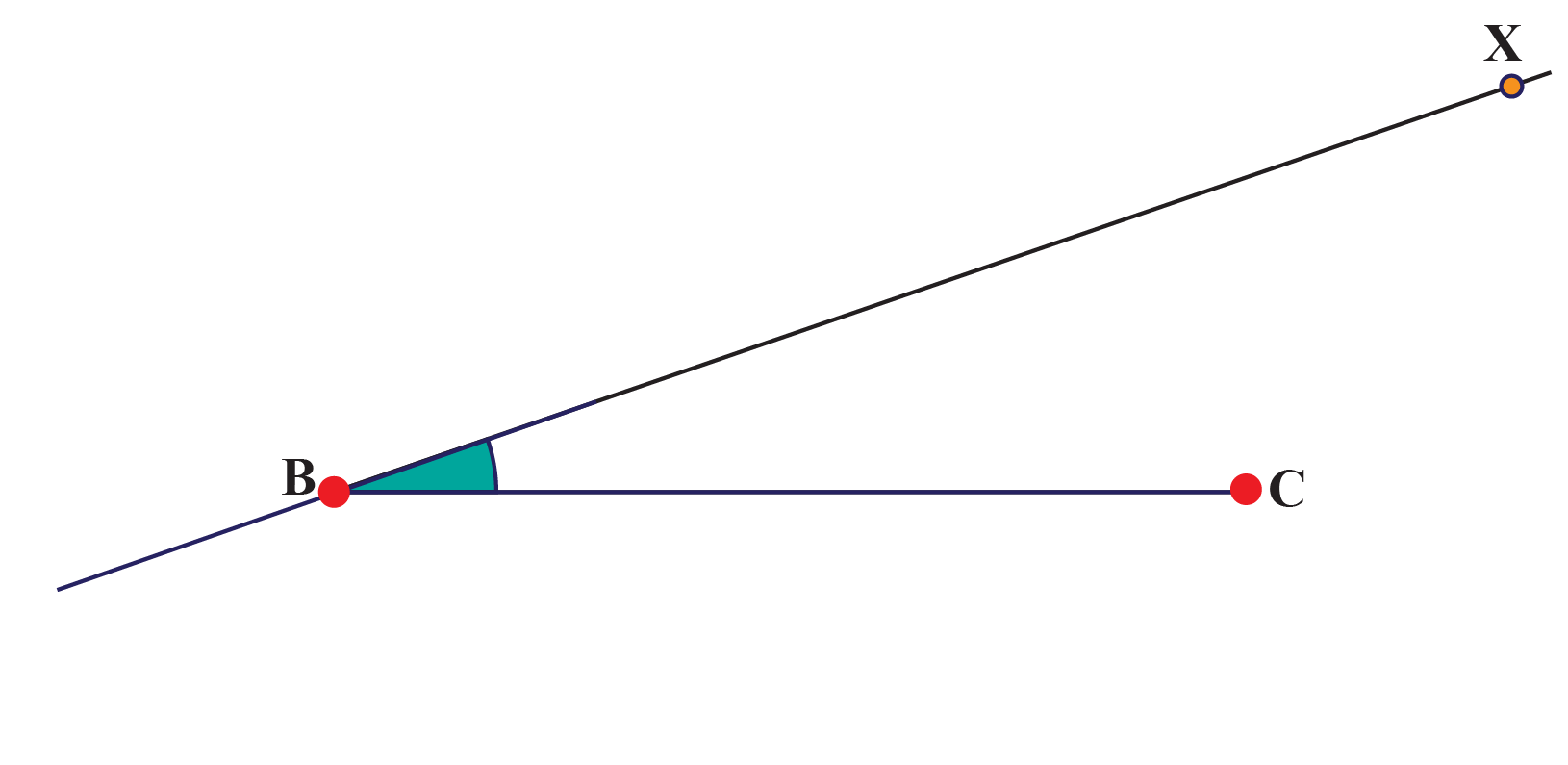Cut off BD = AC – AB on this extended ray: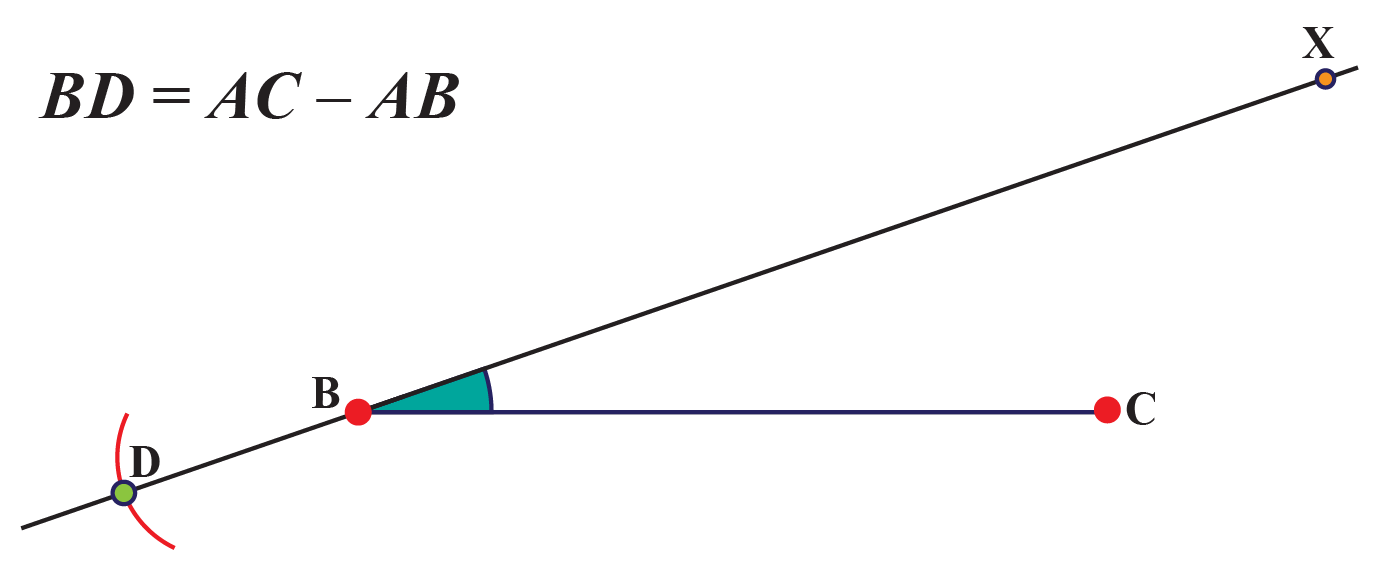Step 3: Join CD. Draw the perpendicular bisector of CD, and let it intersect ray BX at A: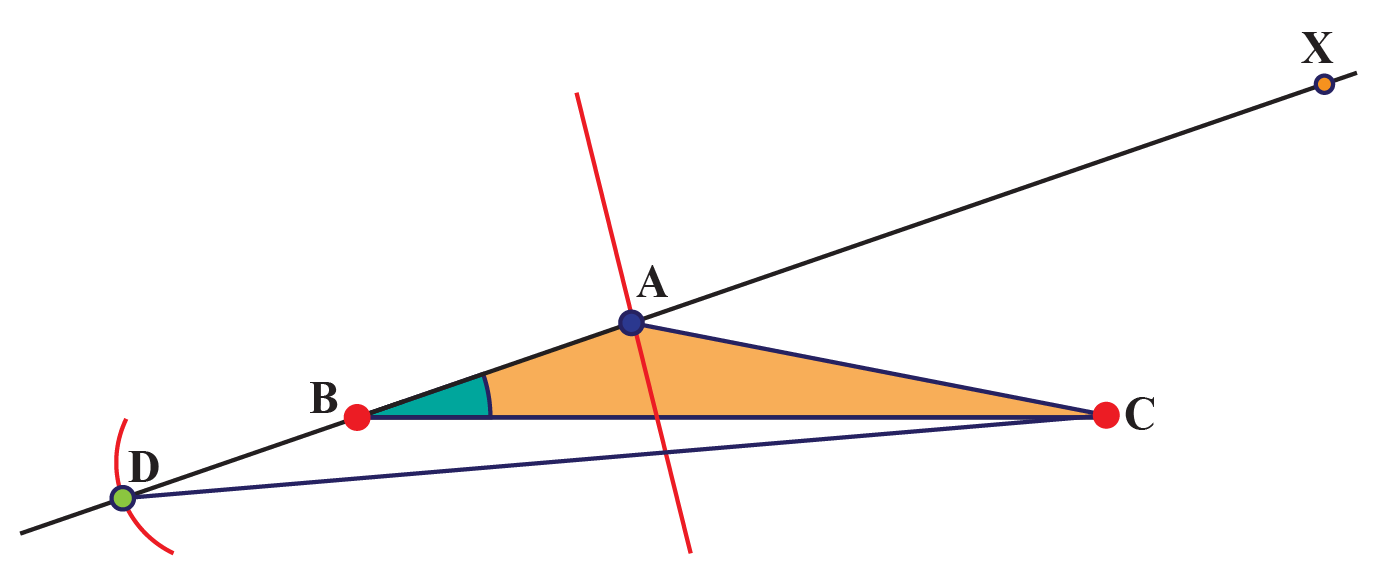$$\Delta ABC$$ is the required triangle. This is because AC = AD (why?) and:

BD = AD – AB = AC – AB

### Method 3

The perimeter and two base angles are given.

We will construct $$\Delta ABC$$, given $$\angle B$$, $$\angle C$$ and AB + BC + CA.

Step 1: Construct a segment PQ = AB + BC + CA, and draw rays PX and QY, inclined to PQ at $$\angle B$$ and $$\angle C$$ respectively.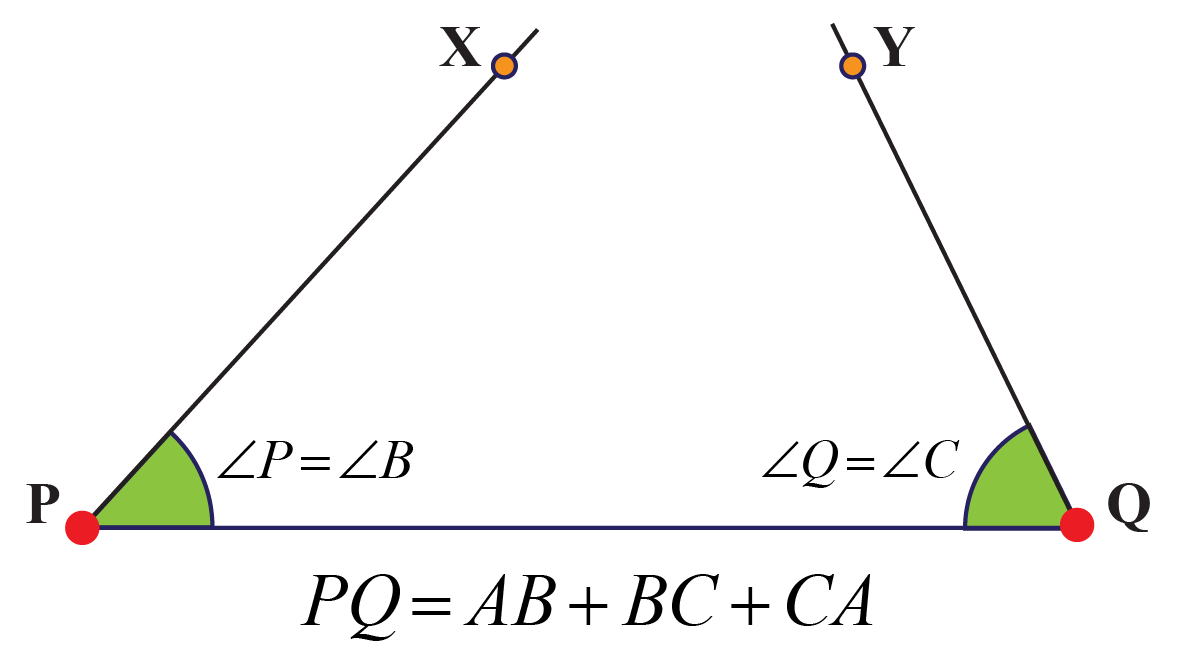Step 2: Draw the angle bisectors of $$\angle XPQ$$and $$\angle YQP$$, and denote their point of intersection by A:Step 3: Draw the perpendicular bisectors of AP and AQ, and let them intersect PQ at B and C respectively: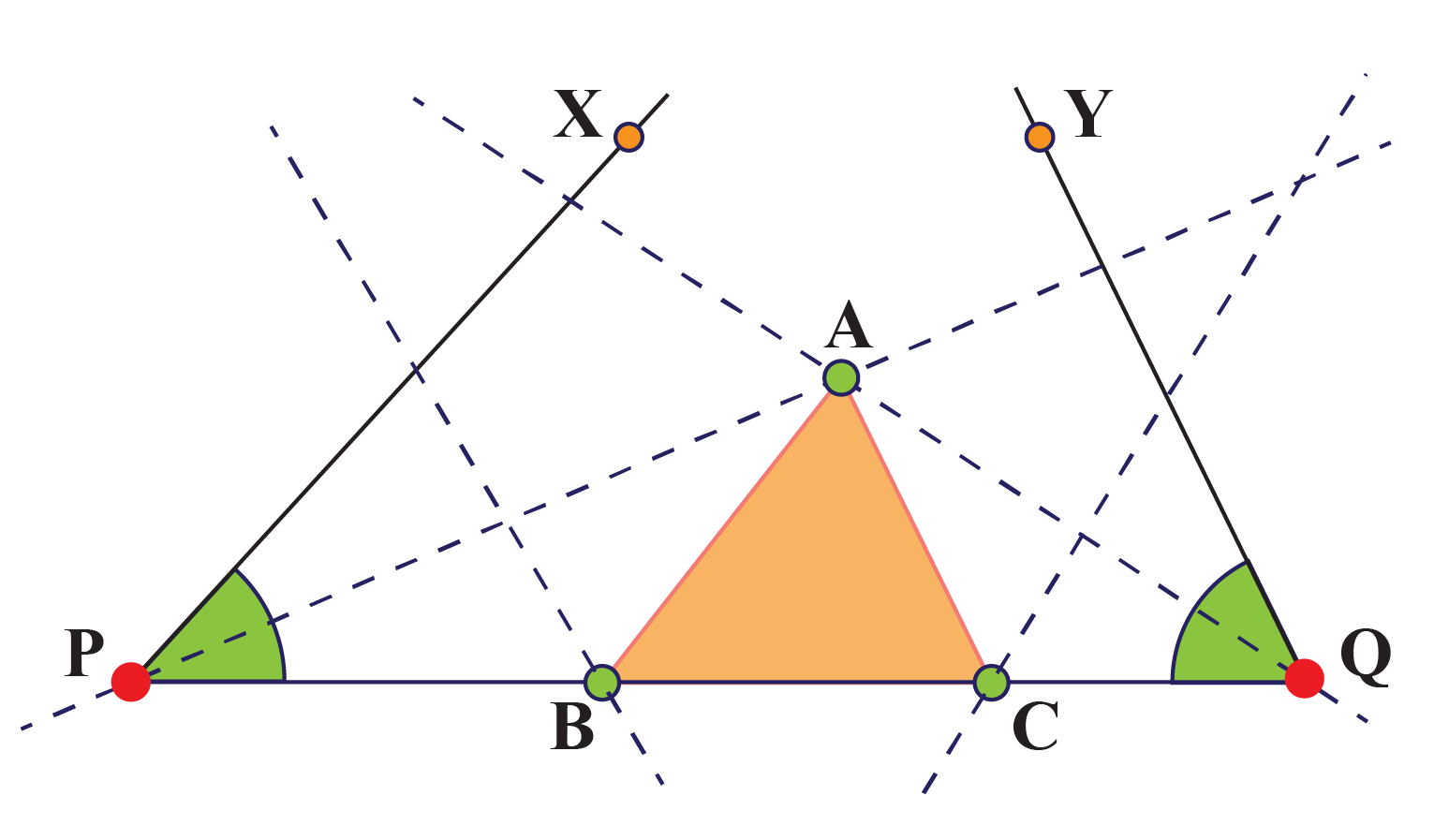$$\Delta ABC$$ is the required triangle. Let us see how:

1. Since B lies on the perpendicular bisector of AP, we have AB = PB. Similarly, AC = CQ, which means that AB + BC + CA = PB + BC + CQ = PQ. This is consistent with our earlier construction.

2. Since $$\Delta ABP$$ is isosceles, we have $$\angle BPA$$= $$\angle BAP$$. Thus, $$\angle ABC$$= $$\angle BPA$$+ $$\angle BAP$$= 2$$\angle BPA$$= $$\angle XPQ$$. Recall that we constructed angle $$\angle XPQ$$ equal to one of the given angles. Thus, $$\angle ABC$$ turns out to have the same value.

3. Similarly, $$\angle ACB$$ will turn out to be the same as $$\angle YQP$$, the other angle we were originally given.Important Notes
1. The triangles can be classified on the basis of angles and sides.
2. You need a minimum of 3 distinct parameters for the construction of any triangle.

## Solved Examples

 Example 1

Mathews told his friend James that he created an obtuse right-angled triangle. James immediately pointed out that it’s not possible. What do you think is the reason?

### Solution

In a right-angled triangle, one angle is always 90o. And we know that the sum of all angles of a triangle is 180o.

So the sum of the other 2 angles must be also equal to 90o, which means both of the angles are less than 90o, so one of them cannot be more than 90. And if none of the angles are more than 90, then it cannot be an obtuse-angled triangle.

So the claim that Mathews saw an obtuse right-angled triangle is false.

 $$\therefore$$ James' claim is correct.
 Example 2

Construct a $$\Delta ABC$$ such that AB = 4 cm, $$\angle A$$ = 60o and the sum of AC and BC is 11 cm.

Solution

The various steps of the construction are outlined below:

Draw AB = 4 cm, and ray AX such that $$\angle XAB$$= 600: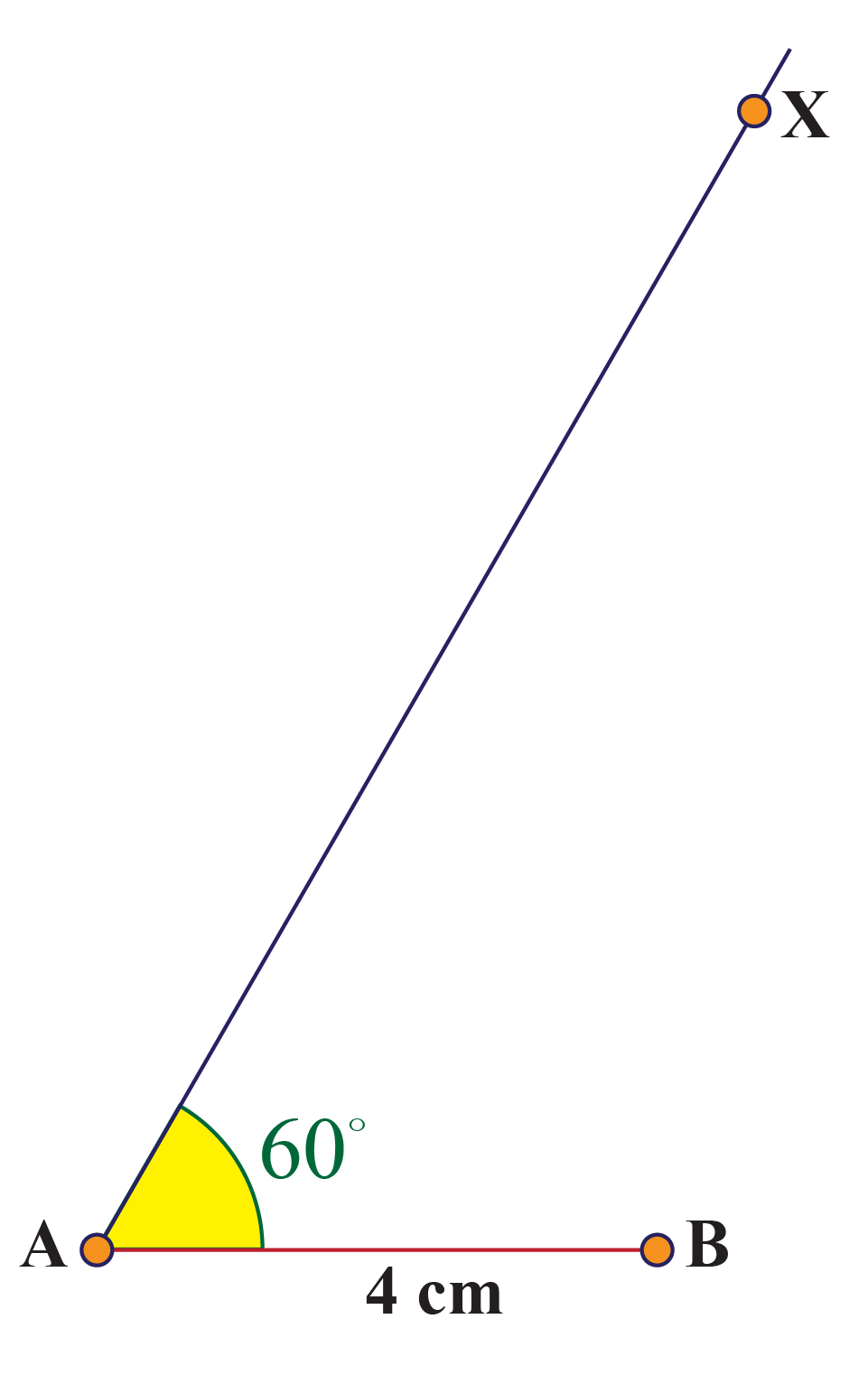Now, cut off AD = 11 cm on AX, join BD and draw the perpendicular bisector of BD. Let it intersect AX at C: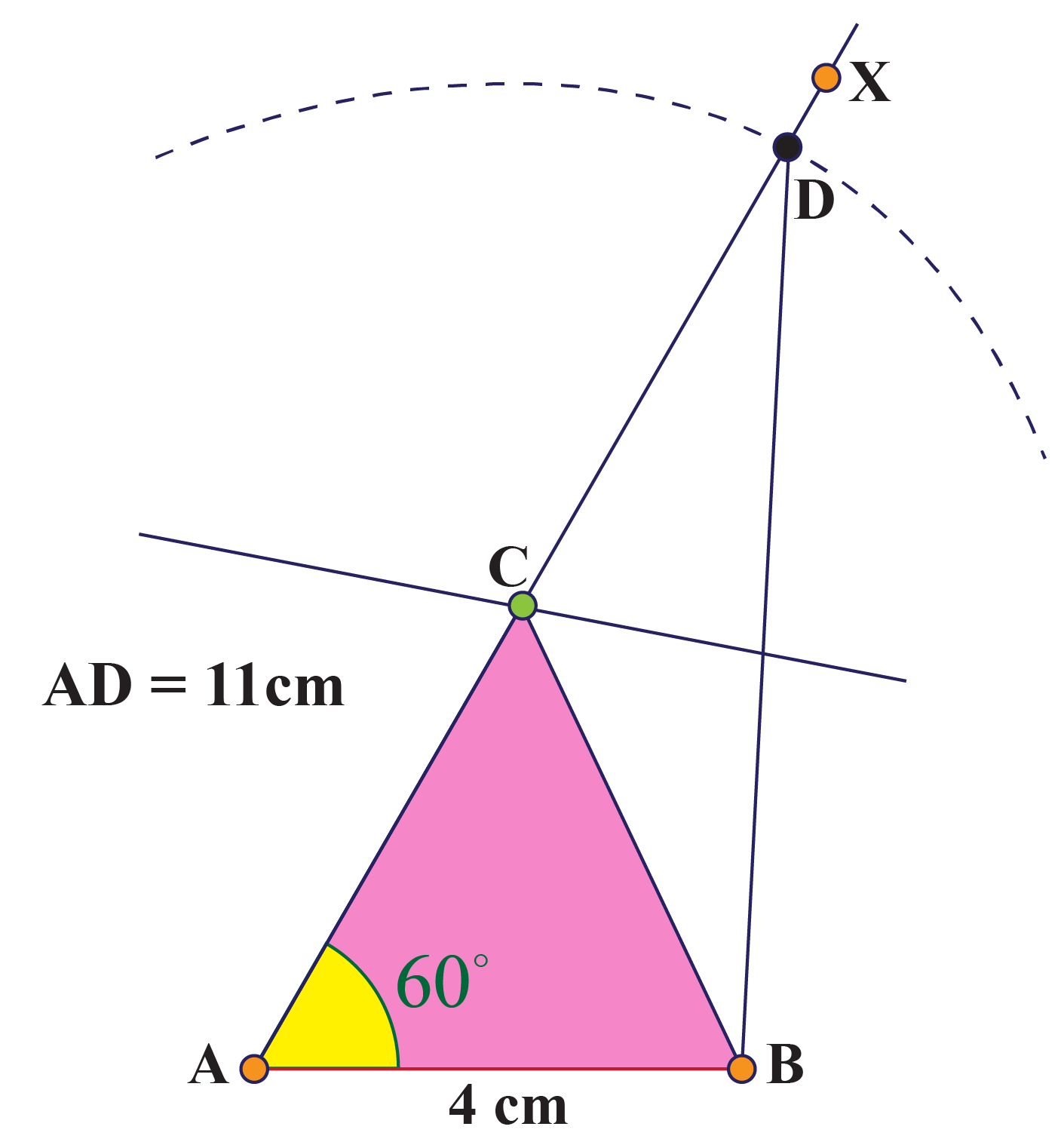$$\Delta ABC$$ is the required triangle. Justify this construction without referring to the earlier proof.

 $$\therefore$$ The triangle is constructed.
 Example 3

Construct a $$\Delta ABC$$ such that $$\angle B$$ = 600, $$\angle C$$ = 450 and the perimeter of the triangle is 13 cm.

Solution

Draw a segment PQ = 13 cm. Draw rays PX and QY such that $$\angle XPQ$$= 600 and $$\angle YQP$$= 450: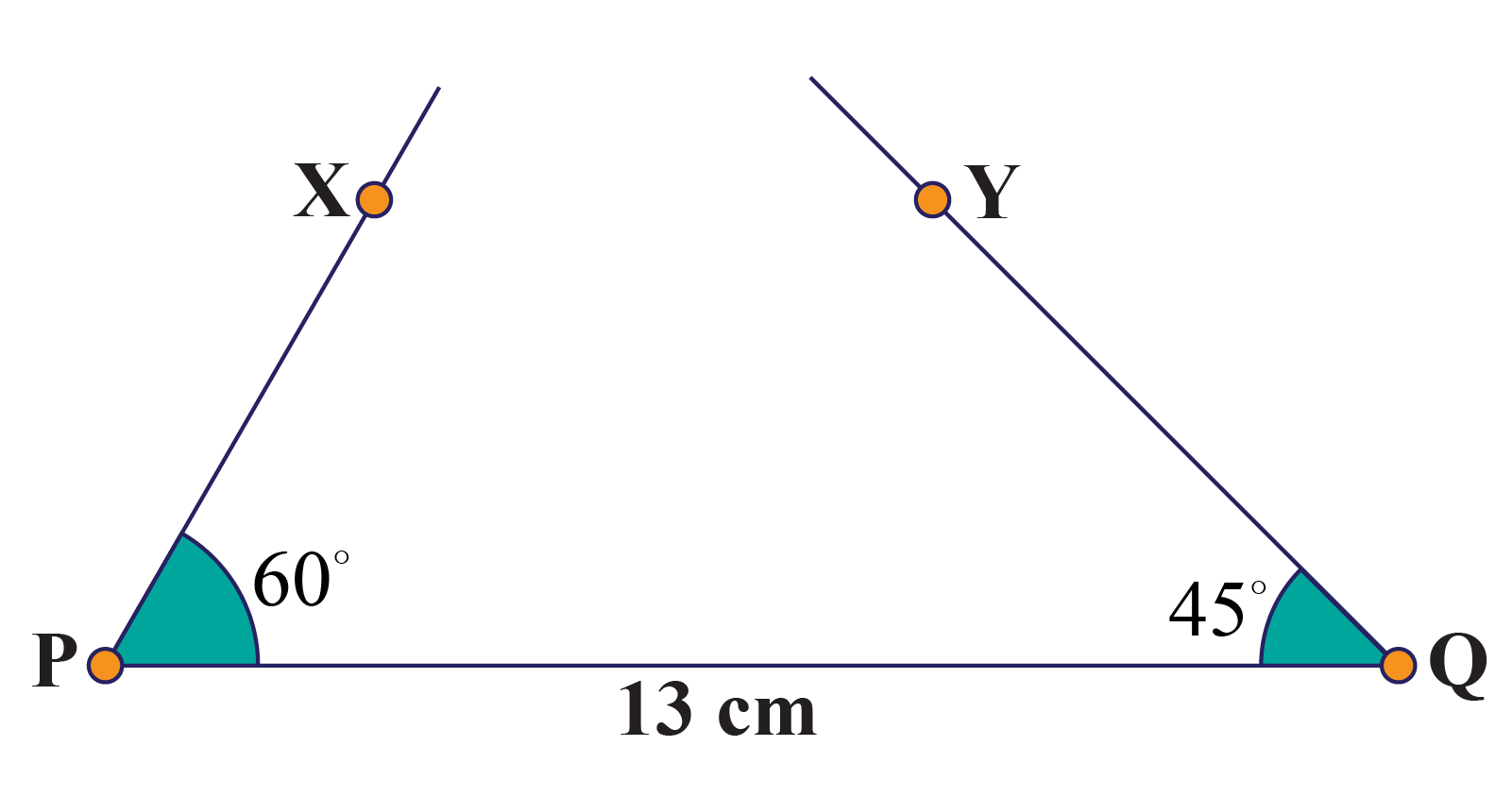Draw the angle bisectors of these two angles, and denote their point of intersection by A: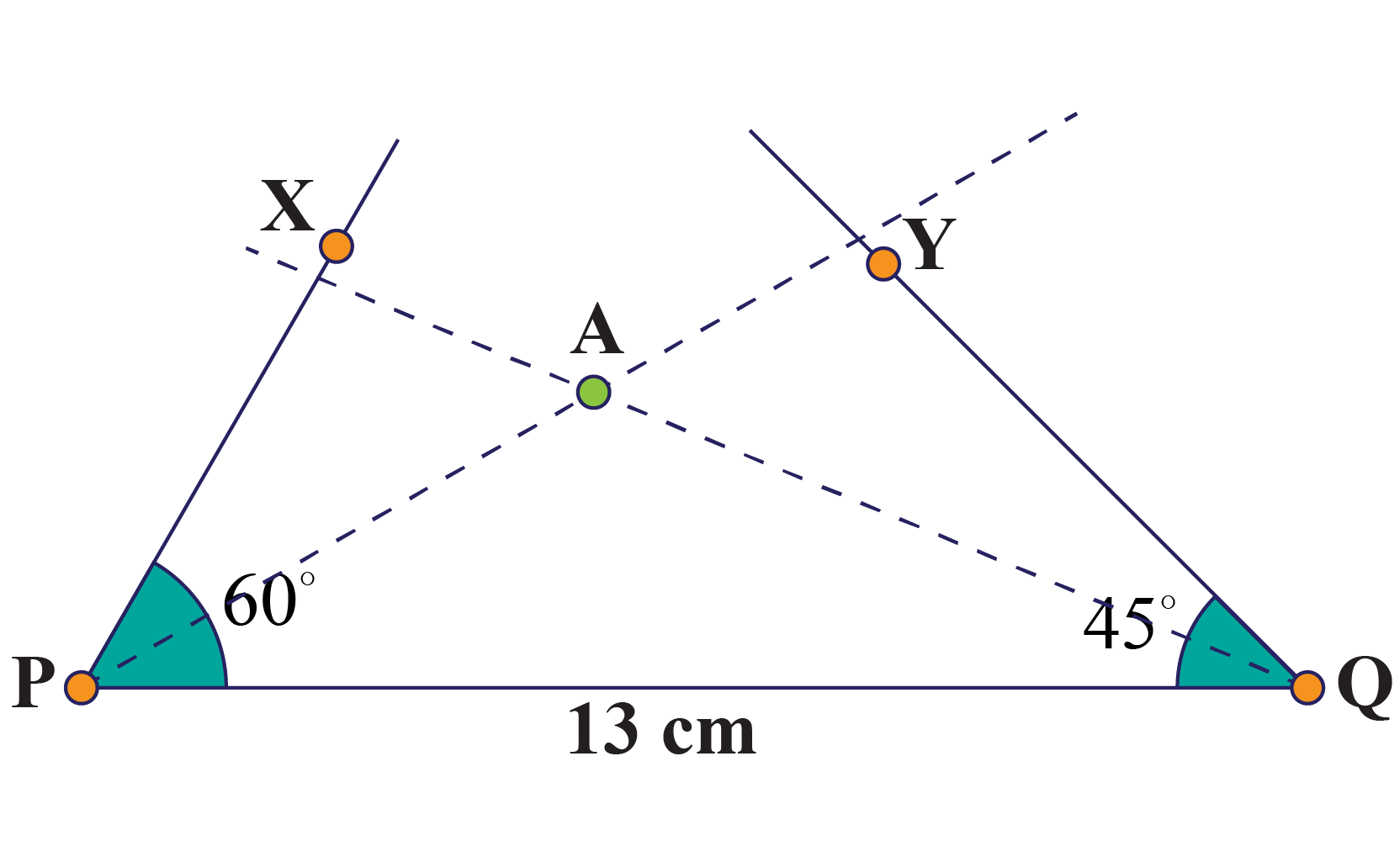Draw the perpendicular bisectors of AP and AQ, and denote their points of intersection with PQ by B and C: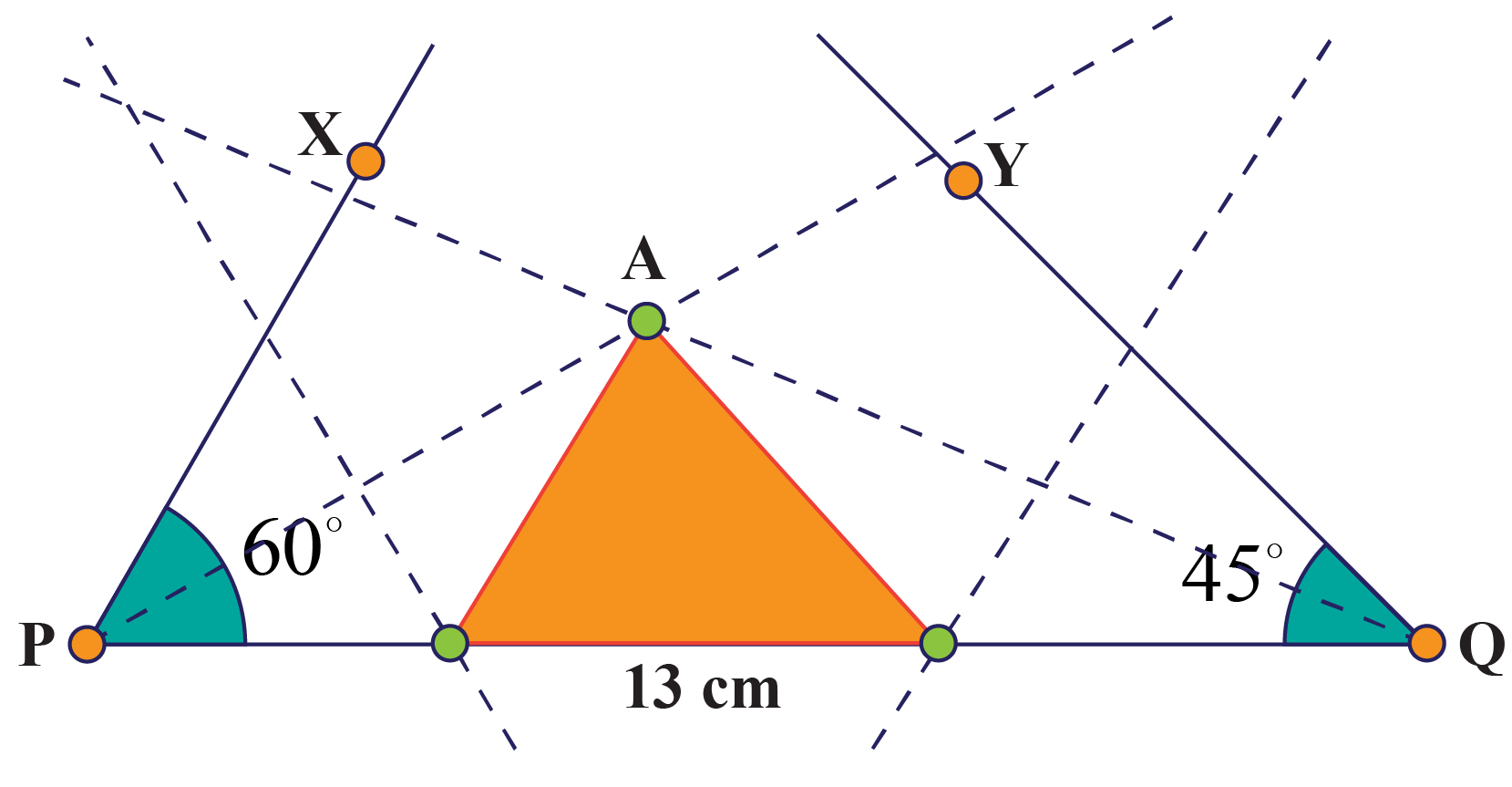$$\Delta ABC$$ is the required triangle.

 $$\therefore$$ The triangle is constructed.Challenging Questions

Can you construct a triangle whose sides are in arithmetic progression with a difference of 6 units and perimeter 72 units?

## Interactive Questions

Here are a few activities for you to practice. Select/Type your answer and click the "Check Answer" button to see the result.

## Let's Summarize

The mini-lesson targeted the fascinating concept of the Construction of a Triangle. The math journey around the Construction of a Triangle starts with what a student already knows, and goes on to creatively crafting a fresh concept in the young minds. Done in a way that is not only relatable and easy to grasp but will also stay with them forever. Here lies the magic with Cuemath.

At Cuemath, our team of math experts is dedicated to making learning fun for our favorite readers, the students!

Through an interactive and engaging learning-teaching-learning approach, the teachers explore all angles of a topic.

Be it worksheets, online classes, doubt sessions, or any other form of relation, it’s the logical thinking and smart learning approach that we, at Cuemath, believe in.

### How do you construct a triangle accurately?

Given the constraints of sides and angles, we can construct a unique triangle if at least 3 of its sides and angles are known to us.

### How do you draw a triangle step by step?

We can use a ruler, a compass, and a pencil, to properly draw a triangle starting from its base, then drawing the other 2 of its side, with the specified angles.

### What are the types of triangles?

The triangles can be classified on different bases.

Based on lengths of sides there are – equilateral, isosceles, and scalene
Based on angles there are – acute-angled, right-angled, and obtuse-angled.

### What are the angles of a triangle?

Angles of a triangle are formed when 2 sides of a triangle touch each other. Angles can be measured in degrees or radians and are an important and unique property of any triangle.

### Can we construct a triangle with 3 angles?

Yes, a triangle is constructed only with 3 angles.

### Which angles can make a triangle?

Any angles that sum up to 180 degrees can make a triangle.

grade 9 | Questions Set 1